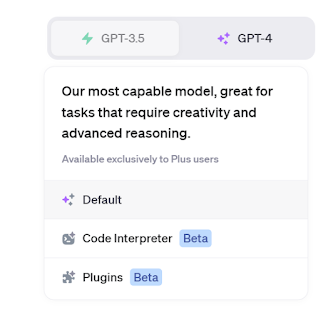## Friday 30 December 2016

### Neuron Controlled Edge follower updated

In the last post experimentation with an artificial neuron controlling the Bit:Bot to follow the edge of a line (it follows the left-hand side of the line).

More details can be found in the previous post.The neurons (well two separate ones, S1 and S2) are produced using weighted sums - summing the weights x inputs [ right-hand sensor (rs) and left-hand sensor (ls)] plus a bias for each neuron in this case w and w.
net=w+w*rs+w*ls
net2=w+w*rs+w*ls

If weighted sum >=0 then its output 1 otherwise 0

What actual causes S1 to be either 1 or 0 is all defined by a set of weights w (three for the first neurone, S1,  three for S2).

w=[0,-1,1,-1,1,-1]

Modifications to the code in the last post have been around fine tuning the values in converting the outputs of the two neurons S1 and S2 into actions as shown below.
if s1==1 and s2==1:
forward(20)
elif s1==0 and s2==1:
forward(15)
right_turn(25)
elif s1==1 and s2==0:
forward(15)
left_turn(25)
elif s1==0 and s2==0:
backward(5)

The functions for forward, right_turn, etc are defined elsewhere.

To change the function of the system, change the values in wThe complete code is shown below.

Code
from microbit import *
import neopixel, random, array

w=[]

def forward(n):
pin0.write_analog(551)
pin8.write_digital(0)
pin1.write_analog(551)
pin12.write_digital(0)
sleep(n)

def backward(n):
pin0.write_analog(551)
pin8.write_digital(1)
pin1.write_analog(551)
pin12.write_digital(1)
sleep(n)

def right_turn(n):
pin0.write_analog(511)
pin8.write_digital(0)
pin1.write_analog(511)
pin12.write_digital(1)
sleep(n)

def left_turn(n):
pin0.write_analog(551)
pin8.write_digital(1)
pin1.write_analog(551)
pin12.write_digital(0)
sleep(n)

w=[0,-1,1,-1,1,-1]

while True:

net=w+w*rs+w*ls
net2=w+w*rs+w*ls

if net>=0:
s1=1
else:
s1=0

if net2>=0:
s2=1
else:
s2=0

if s1==1 and s2==1:
forward(20)
elif s1==0 and s2==1:
forward(15)
right_turn(25)
elif s1==1 and s2==0:
forward(15)
left_turn(25)
elif s1==0 and s2==0:
backward(5)

Video of it in action:

Please feel free to use the code and improve on it, and I would especially welcome the seeing the improvement through the comments.

All opinions in this blog are the Author's and should not in any way be seen as reflecting the views of any organisation the Author has any association with. Twitter @scottturneruon

#### 1 comment:

1.This comment has been removed by a blog administrator.

### ChatGPT, Data Scientist - fitting it a bit

This is a second post about using ChatGPT to do some data analysis. In the first looked at using it to some basic statistics  https://robots...